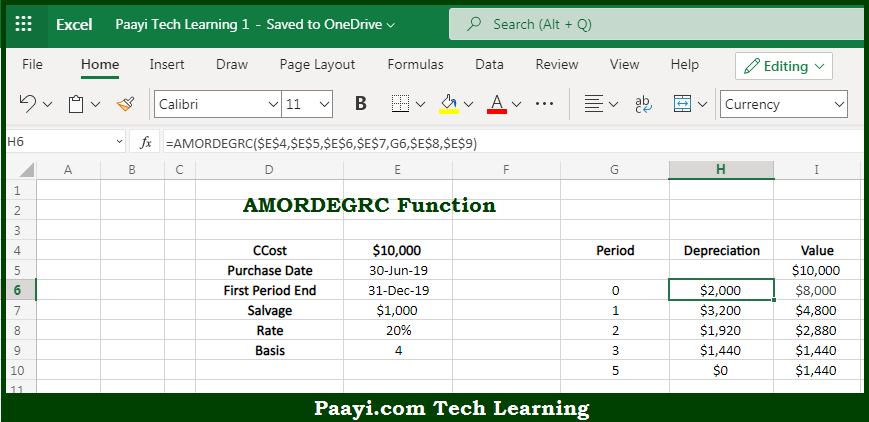# Learn How to Use Microsoft Excel AMORDEGRC Function

Written by | 0 Comments | 660 Views

In this article, you will learn how to use the Microsoft Excel AMORDEGRC function and its prime function in Microsoft Excel. You will also get to know the Microsoft Excel AMORDEGRC function return value and syntax with the help of some examples.

Microsoft Excel AMORDEGRC Function

The main purpose of the Microsoft Excel AMORDEGRC function is to get the depreciation for the accounting period coefficient. That implies, with the help of the AMORDEGRC function you can able to return the depreciation for a given accounting using a depreciation coefficient determined by asset life. So, with the help of the AMORDEGRC function, you can able to get the depreciation for the accounting period coefficient.

Return Value of AMORDEGRC Function

The return value will be the depreciation in the given period.

Syntax of AMORDEGRC Function

=AMORDEGRC(cost, purchase, first, salvage, period, rate, [basis])

Where the arguments:

• cost: This is the asset cost.
• purchase: This is the asset purchase date.
• first: This is the first-period end date.
• salvage: This is the asset salvage value.
• period: This is the period for which you want to calculate the depreciation.
• rate: This is the rate of depreciation.
• basis: This is the day count basis, default is 0 (optional).

How to Use Microsoft Excel AMORDEGRC Function?So we know that Microsoft Excel AMORDEGRC function you can able to get the depreciation for the accounting period coefficient. That implies, with the help of the AMORDEGRC function you can able to return the depreciation for a given accounting using a depreciation coefficient determined by asset life. So, with the help of the AMORDEGRC function, you can able to get the depreciation for the accounting period coefficient.